# Rhombus problem

Perimeter of the rhombus is 204 dm and it area is 2244 dm2. Calculate the height of the rhombus.

Result

h =  44 dm

#### Solution:Leave us a comment of example and its solution (i.e. if it is still somewhat unclear...):Be the first to comment!## Next similar examples:

1. Rhombus HP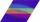Calculate area of the rhombus with height 24 dm and perimeter 12 dm.
2. Acreage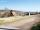Plot has a diamond shape, its side is 25.6 m long and the distance of the opposite sides is 22.2 meters. Calculate its acreage.
3. Strip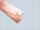From 5.9 cm wide strip should be cut rhombus with area 28 cm2. How long will be its side?
4. Write 3Write a real world problem involving the multiplication of a fraction and a whole number with a product that is between 8 and 10 then solve the problem
5. RectangleThe rectangle area is 182 dm2, its base is 14 dm. How long is the other side? Calculate its perimeter.
6. Odometer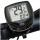The odometer is driven by rotation of the wheel whose diameter is 65 cm. After how many rotations the wheel turns counter to next kilometer?
7. Right triangleRight triangle legs has lengths 630 mm and 411 dm. Calculate the area of this triangle.
8. Fractions 3Calculate 1/9 of 27:
9. Double 5Peter was thinking of a number. Peter doubles it and gets an answer of 8.6. What was the original number?
10. Scouts 4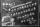4/7 of the students in a school are boys. If 3/8 of the boys are scouts, how many scouts are there in a school of 1878 students?
11. Pie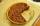Vili ate three pieces of pie . If it piece is 1/8 how much pie did he eat?
12. What isWhat is the value of the smaller of a pair of numbers for which their sum is 78 and their division quotients are 0.3?
13. Pizza 5You have 2/4 of a pizza and you want to share it equally between 2 people how much pizza does each person get?
14. Date game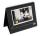Let now is Wednesday. What day is after 248 days? Write the result as a number: 1=Monday, 2=Tuesday, 3=Wednesday, 4=Thursday, 5=Friday, 6=Saturday, 7=Sunday?
15. Crates 2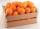One crate will hold 50 oranges. If Bob needs to ship 932 oranges, how many crates will he need?
16. Product of two fractionsProduct of two fractions is 9 3/5 . If one of the fraction is 9 3/7. Find the other fraction.
17. Fractions 4How many 2/3s are in 6?